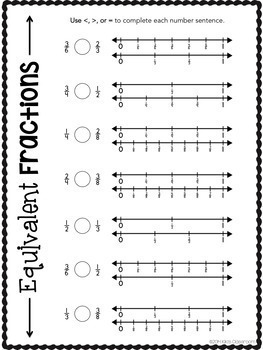# Equivalent Fractions On a Number Line 3.NF - Comparing Fractions WorksheetsSubject
Resource Type
File Type

Zip

(34 MB|46 pages & flipbook)
Product Rating
Standards
• Product Description
• StandardsNEW

These printable worksheets cover ALL of the third grade 3.NF standards, presenting concepts "every which way" to build mastery and deepen understanding. This bundle includes 27 pages of printables, plus materials for a “Ways to Show ½” Sorting Flip Book—perfect for 3.NF.A.3.B!

The introduction of fractions in third grade represents a big conceptual leap, so providing a strong foundation is essential. This pack is designed to give kids a range of experiences with fractions, number lines, and fraction models. Care has been taken to include models in a variety of shapes, and in a variety of orientations. All work is based on the CCSS-specified denominators for third grade: 2, 3, 4, 6, and 8.

This Print & Go BUNDLE is useful as:

• skills practice as you encounter these fractions concepts in your math curriculum,

• practice for early finishers,

• small group work,

• homework, or

• in math centers.

Blackline “My Fractions Packet” cover pages are included for each of the 3 fractions packs, should you choose to use them as student packets.

★ This bundle is available at an even deeper discount, in the 3rd Grade Math Essentials MEGA BUNDLE! ★

••••••••••••••••••••••••••••••••••••••••••••••••••••••••••••••••••••••••••••••••••••••

In order to build their skills, children are asked to:

recognize equal parts within a range of “wholes”;

• to estimate to partition a variety of polygons into fractional parts;

• to identify fractions shown within a variety of polygons;

• to write fraction names shown in a variety of polygons;

• to regcognize named fractions shown in a series of polygons;

• to name fractional parts given a real-world context (products at the grocery store);

• to match fractions with corresponding points and line segments shown on number lines;

• to write fraction names for points and line segments shown on number lines;

• to draw points and line segments on unlabeled number lines;

• to estimate to partition unlabeled number lines and label given fractions;

• to use number lines in a variety of ways to find equivalent fractions;

• to plot fractions on unlabeled number lines;

• to complete equations using equivalent fractions,

• to order fractions with like denominators;

• to find the greatest fraction in a series of pairs;

• to sort equivalent fractions into groups;

• to order a series of fractions with different denominators from least to greatest;

• to write mixed numbers for fraction models;

• to match mixed number fraction models to their fraction names; and

• to provide an extended response explaining why two fractions are equivalent.

••••••••••••••••••••••••••••••••••••••••••••••••••••••••••••••••••••••••••••••••••••••

This money-saving BUNDLE includes 3 Fractions: Print & Go Packs—each designed to meet a Grade 3 CCSS Math Standard for FRACTIONS—plus the “Ways to Show ½” Fraction Sort Flip Book.

Fractions Print & Go Pack: 3.NF.A.1

Fractions Print & Go Pack: 3.NF.A.2

Fractions Print & Go Pack: 3.NF.A.3

"Ways to Show 1/2" Fraction Sort Flip Book

••••••••••••••���••••••••••••••••••••••••••••••••••••••••••••••••••••••��••••••••••••••••

You may also be interested in these Grade 3 CCSS-aligned math products:

Multiplication BUNDLE

Multiplication Print & Go Pack

Merry, Merry Multiplication

Geometry Game: "I Have...Who Has...?"

Beat the Clock: Math Fact Practice Bundle

••••••••••••••••••••••••••••••••••••••••••••••••••••••••••••••••••••••••••••••••••••••

Compare two fractions with the same numerator or the same denominator by reasoning about their size. Recognize that comparisons are valid only when the two fractions refer to the same whole. Record the results of comparisons with the symbols >, =, or <, and justify the conclusions, e.g., by using a visual fraction model.
Express whole numbers as fractions, and recognize fractions that are equivalent to whole numbers. Examples: Express 3 in the form 3 = 3/1; recognize that 6/1 = 6; locate 4/4 and 1 at the same point of a number line diagram.
Recognize and generate simple equivalent fractions, (e.g., 1/2 = 2/4, 4/6 = 2/3). Explain why the fractions are equivalent, e.g., by using a visual fraction model.
Understand two fractions as equivalent (equal) if they are the same size, or the same point on a number line.
Explain equivalence of fractions in special cases, and compare fractions by reasoning about their size.
Total Pages
46 pages & flipbook
N/A
Teaching Duration
N/A
Report this Resource to TpT
Reported resources will be reviewed by our team. Report this resource to let us know if this resource violates TpT’s content guidelines.\$9.25
List Price:
\$13.00
You Save:
\$3.75